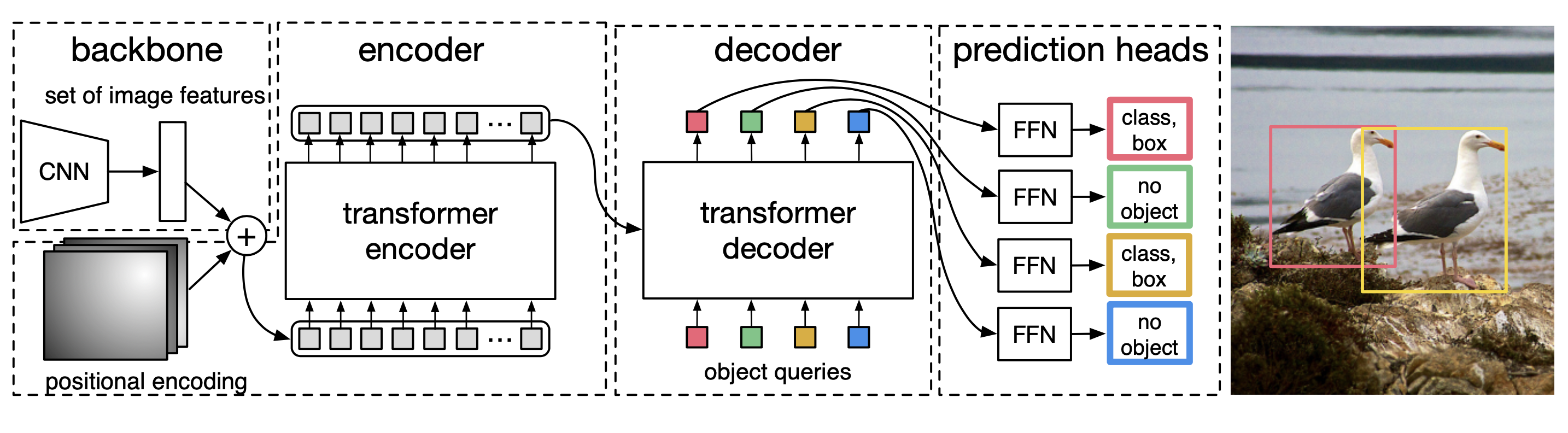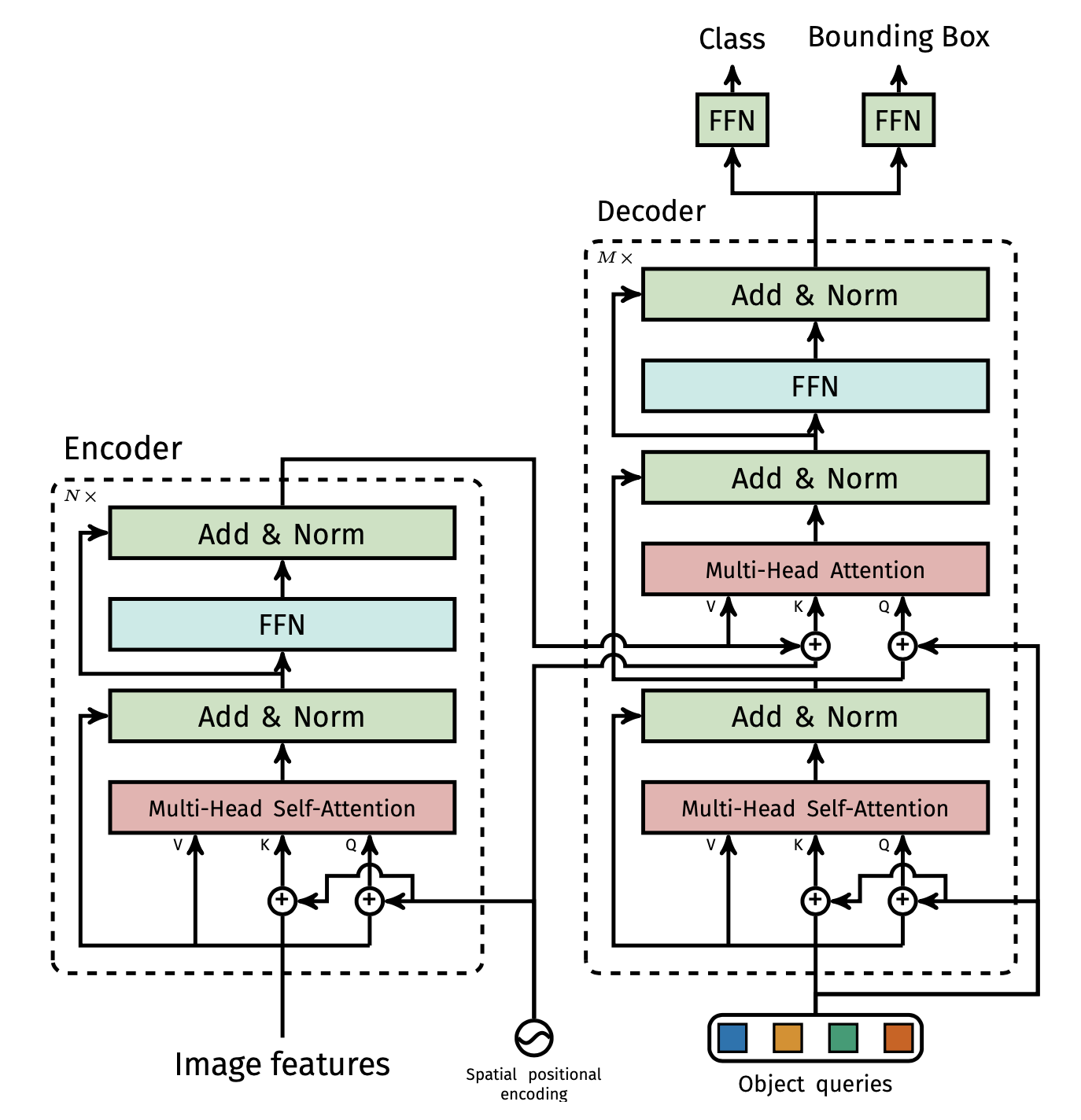# End-to-End Object Detection with Transformers

DETR still uses CNN for feature extration and then use transformer to capture context of objects (boxes) in images. Compared with previous object detection model, e.g., MaskRCNN, YOLO, it doesn’t need to have anchor and nonmaximal suppression, which is achived by transformer. Besides DETR could be directly applied for panoptic segmentation (joint semantic segmentation and instance segmentation).

# Network Architecture

DETR could be visualized as below:• backbone can be any (convolution) neutral network, e.g., ResNet50 which extracts feature from $$H_0\times W_0\times 3$$ image to $$H\times W\times C$$ feature tensor, where paper recommends $$H=\frac{H_0}{32}$$, $$W=\frac{W_0}{32}$$ and C=2048
• position encoding provides spatial coordinate between the feature vector. The paper proposes to use $$\frac{d}{2}$$ sine and cosine coordianate as different frequencies.
• position enoding is applied to each attention layer
• position encoding could be learned from data as well, which will be shared to all attention layers
• spatial encoder contributes 7.8 AP
• Encoder encodes the input feature from $$d\times HW$$ into output as $$d\times HW$$:
• First $$1\times 1$$ convolution is applied to extra feature to tranform the feature from $$H\times W\times d$$ and reshaped to $$d\times HW$$ feature vector;
• The spatial encoding is then added to the feature vector;
• The encoder incldues several (6) encoder layers, each of which contains a multi-head self-attention module and feedforward network (FFN).
• ablation study shows increasing # encoder layers from 0 to 12, AP increases consistently
• ablation study shown that without encoder, overall AP drops by 3.9 points, with a more significant drop of 6.0 AP on large objects
• Decoder transforms N object queries at d dimension into N output embedding at d dimension:
• object queries are learned from during training steps;
• apply encoder-decoder ove these embeddings enables reasoning about all objects all together using pair-wise relationships;
• the paper used 6 decoder layers
• Both AP and AP50 improve after every layer, totalling into a very significant +8.2/9.5 AP improvement between the first and the last layer.
• Feedback forward network (FFN) converts each of d-dimensional output embmedding into class labels (via softmax) and normalized object bounding boxes (object center and box size). FFN is a 3-layer perceptron with ReLU activate function and hidden dimension is d.
• a background class is added
• FFN is applied after each decoder layer. This contributes to 2.3 AP

The architecture of transformer is shown as below:# Loss Functions

DETR estimates N object detections for each image, where N is set to be larger than max possible objects in the images. N is fixed as part of network. DETR relies on Hungarian loss, which measures the an optimal bipartite matching between estimation ($$\hat{y}$$) and ground truth (y) objects and then optimize object-specific losses.

$\ell(y,\hat{y})=\sum_i^N[-\log{p_{\sigma(i)}(c_i)}+\mathbb{1}_{c_i\neq\emptyset}\ell_{box}(b_i,b_{\sigma(i)})]$

Each object $$y_i$$ is represented by object labels $$c_i$$ (with $$\emptyset$$ as background or non-class) and bounding box $$b_i$$ ($$\mathbb{R}^4\in[0,1]$$). $$\sigma(i)$$ defines the mapping of estimation to ground truth. For estimation labeled as the background the class label loss is down-weighted by 10.

$\sigma:\min_\sigma{\sum_i^N\ell_{match}(y_i,\hat{y}_{\sigma(i)}}$

For bounding box loss, the paper proposed to use linear combination of $$\ell_1$$ loss and generalized IoU loss, which is scale-invariant:

$\ell_{box}(b_i,b_{\sigma(i)})=\lambda_{iou}\ell_{iou}(b_i,b_{\sigma(i)})+\lambda_{\ell_1}\lVert b_i-b_{\sigma(i)}\rVert_1$

$$\lambda_{iou}=2$$ and $$\lambda_{\ell_1}=5$$. Ablation study indicates generalized IoU loss contributes 4.8 AP while l1 loss contributes 0.7 AP.

# Implementation Details

The paper trained DETR with AdamW  setting the initial trans- former’s learning rate to 10−4, the backbone’s to 10−5, and weight decay to 10−4. All transformer weights are initialized with Xavier init, and the backbone is with ImageNet-pretrained ResNet model from torchvision with frozen batchnorm layers.

The paper used scale augmentation, resizing the input images such that the shortest side is at least 480 and at most 800 pixels while the longest at most 1333. To help learning global relationships through the self-attention of the encoder, we also apply random crop augmentations during training, improving the per- formance by approximately 1 AP. Specifically, a train image is cropped with probability 0.5 to a random rectangular patch which is then resized again to 800-1333. The transformer is trained with default dropout of 0.1.

Written on March 7, 2021